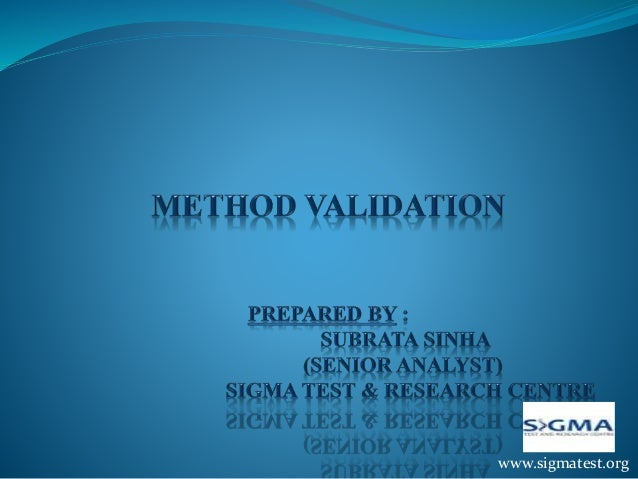Successfully reported this slideshow.
We use your LinkedIn profile and activity data to personalize ads and to show you more relevant ads. You can change your ad preferences anytime.Upcoming SlideShare
Loading in …5
×

# Method Validation - Linearity

2,043 views

Published on

Prepared By :
Subrata SInha (Senior Analyst)

Analytical method validation’s superlative characteristic, linearity is comprehensively explained in this slide show. Linearity commonly understood as proportional relationship between two units, in context of method validation, it refers toproportional relationship between test results and concentrations.

Published in: Data & Analytics
• Full Name
Comment goes here.

Are you sure you want to Yes No
Your message goes here• ➤➤ How Long Does She Want You to Last? Here's the link to the FREE report ♥♥♥ https://tinyurl.com/rockhardxx

Are you sure you want to  Yes  No
Your message goes here

### Method Validation - Linearity

1. 1. www.sigmatest.org
2. 2. LINEARITY Definition:- Linearity is a mathematical relationship between two variable quantities (they may be of the same unit), which are directly proportional to each other. Graphically it represents a straight line when plotted against each other. www.sigmatest.org
3. 3. GRAPHICAL PRESENTATION OF LINEARITY y = 10.107x - 2.381 R² = 0.9997 -100 0 100 200 300 400 500 600 700 0 10 20 30 40 50 60 70 Y-axis X-axis Linearity Graph www.sigmatest.org
4. 4. NEEDNESS OF LINEARITY NON –LINEAR APPROCH LINEAR APPROCH END TIME END TIME 25 min 35 min 25 min 45 min 15 min 35 min 15 min 35 min 10 min 20 min 10 min 30 min START TIME START TIME www.sigmatest.org
5. 5. NEEDNESS OF LINEARITY  From the above graphical presentation of non- linear and linear, We can definitely say that in the linear case we need only one variable value and the other variable value can be accurately determined. But in the case of non-linear we cannot accurately (definitely) determine the other variable’s value, even when one of the variable’s value is known. www.sigmatest.org
6. 6. ROLE OF LINEARITY IN ANALYTICAL METHOD VALIDATION The linearity of an analytical method is it’s ability to elicit test results that are directly proportional to the concentration of the analyte in samples within a given range. This is why to check the proportionality of result with concentrations, it is necessary to justify the linearity between LOWER Concentration & UPPER Concentration. www.sigmatest.org
7. 7. DEFINITION OF SOME PARAMETERS RELATED TO LINEARITY  Lower Concentration:- LOQ is the lower concentration i.e. starting point of the linearity in analytical method validation.  Upper Concentration:- Cmax value is the upper concentration of the linearity in analytical method validation. But it may be lower according to available quantity of the analyte in sample.  Cmax is a pharmacokinetics term. It is the maximum (or peak) serum concentration that an analyte achieves in a specified test area of the body after the analyte has been administrated. www.sigmatest.org
8. 8. JUSTIFICATION OF LINEARITY  The strength & direction of the linear relationship between two variable quantities is termed as correlation coefficient. It is denoted by r.  Mathematically it is expressed by r2, i.e. square of r, which is called coefficient of determination. The coefficient of determination (r2) ranges from 0 to 1. The range of r2 denotes how straight the line is.  Accepted value of r2 is NLT 0.95 www.sigmatest.org
9. 9. The End of Linearity Chapter www.sigmatest.org